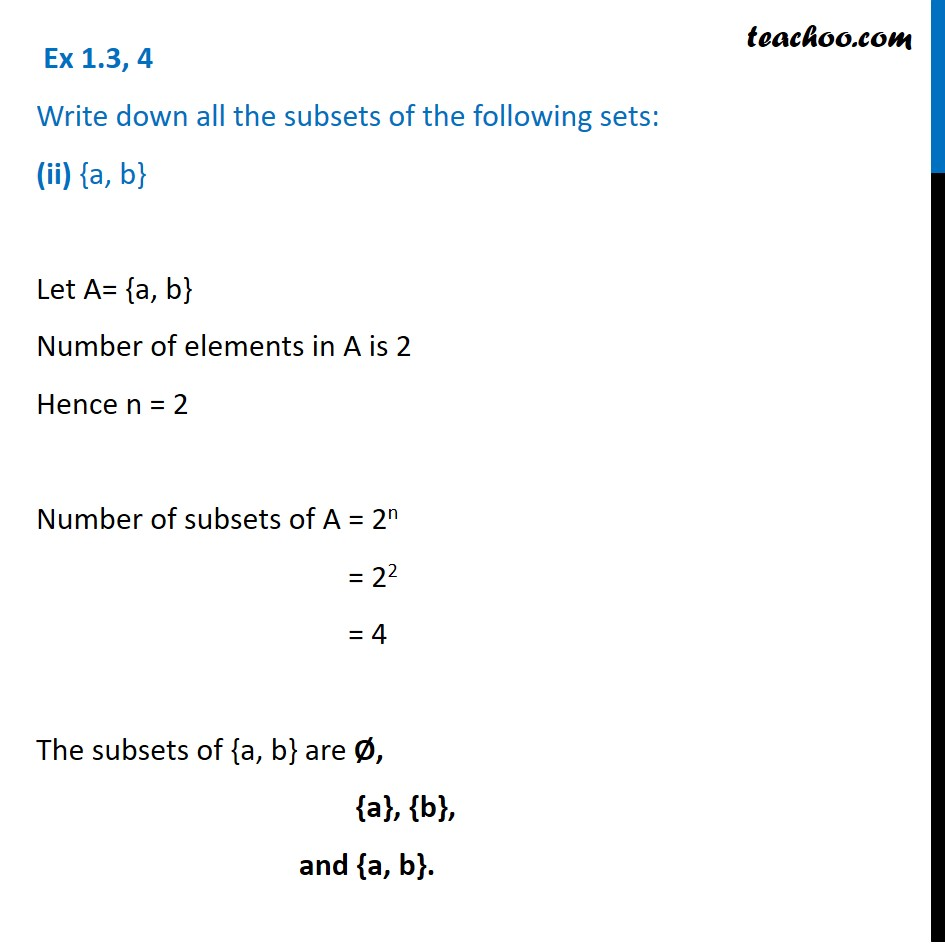Ex 1.3

Chapter 1 Class 11 Sets
Serial order wiseLearn in your speed, with individual attention - Teachoo Maths 1-on-1 Class

### Transcript

Ex 1.3, 4 Write down all the subsets of the following sets: (ii) {a, b} Let A= {a, b} Number of elements in A is 2 Hence n = 2 Number of subsets of A = 2n = 22 = 4 The subsets of {a, b} are Ø, {a}, {b}, and {a, b}.